Home  │ Audio Home Page

Updated March 26, 2011.  See document history at end for details.

## Low-Noise Two-Stage JFET Phono Preamp

This circuit is SPICE verified.  It is only provided for design assistance.  Its final validity awaits someone to prototype it.

Figure 1:  Schematic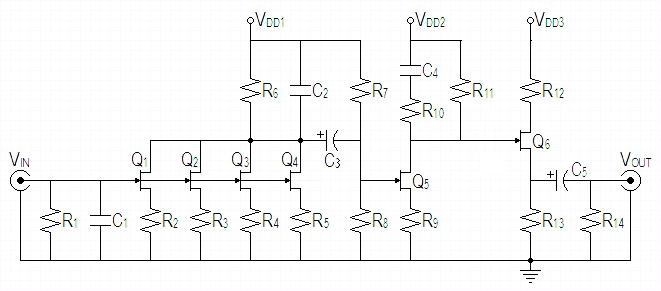Parts List * R1 47kΩ, 1/8V * C1 100pF, metal film Q1-Q5 LSK170A n-ch jfet Q6 LSK170C n-ch jfet R2,R3,R4,R5 56Ω, 5%, 1/8W R6 2kΩ, 1%, 1/2W ** C2a 39nF, 1% plastic film ** C2b 2.7nF, 1% plastic film C3 3.9µF polyethylene R7 10MΩ, 1%, 1/8W
 R8 442kΩ, 1%, 1/8W R9 1.1kΩ, 5%, 1/2W R10 2kΩ, 1%, 1/8W ** C4a 160nF, 1% plastic film ** C4b 16nF, 1% plastic film R11 18.2kΩ, 1%, 1/8W R12 2.4kΩ, 5%, 1/2W R13 2.4kΩ, 5%, 1/2W R14 100kΩ, 5%, 1/8W C5 10uF polyethylene or 180µF electrolytic, see text
* Default values.  R1 and C1 should be calculated to match cartridge LCR per article Phono Termination Calculations and Calculator.
** Capacitor pairs are connected in parallel.
Verify parts list to final SPICE model.

#### Initial Design Decisions

• Design approach is to use higher voltage power supply to allow larger gain with conventional class A circuits.
• Want 5mVRMS to produce 1VRMS at output at 1kHz.  This amounts to 46dB of gain at 1kHz.  Most gain references and calculations are relative to 66dB of gain below 50Hz.  Altered gain goal to standard RIAA 40dB of gain at 1kHz to lower distortion.
• Initially choose 48V power supplies because of unsatisfactory results with 40V supply in prior design cycle.
• Q1-Q5 are LSK170A n-ch jfet (chosen for excellent 1nV/√Hz noise specification).  Use A grade for more possible gain.
• Desireable self bias (Vg-dc = 0V, all bias set by source resistor) on first stage is allowed by small input signal. (Signal will clip if peak is greater than  Vp0 of -0.4025156V; distortion considerations require even more headroom.)
• That a larger signal drives second stage requires more bias than self bias would allow.  Use gate biasing for second stage.
• I chose four devices for stage one because multiple parallel devices devices increase S/N by sqrt(# of devices).  In this case halving the effective noise in the source circuit and to some extent overall.
• Q6 is LSK170C n-ch jfet.  Biasing concerns disadvantage MOSFET here.
• Because of possibility of negative feedback from buffer stage back to gain stage through power supply, I decided to recommend three power supply taps.
 Figure 2:  Stage with 75µS pole (red curve) will boost signal out of noise quicker in first stage than one with 3180µS pole and  318µS zero (black curve).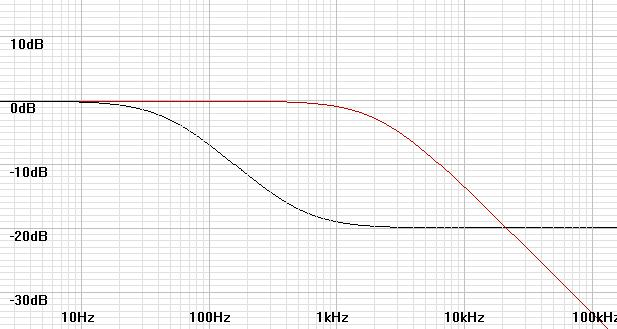#### Gain Stage Design

As an Initial observation, a higher gate bias in the second stage forced by the gain of the first stage would cause the first stage to bear a greater share of the overall gain.

 Figure 3:  LSK170A SPICE model for reference .MODEL LSK170A NJF + BETA   = 0.0378643       VTO    = -0.4025156      LAMBDA = 4.783719E-3        + IS     = 3.55773E-14      + RD     = 10.6565         RS     = 6.8790487                    + CGD    = 3.99E-11        CGS    = 4.06518E-11        + PB     = 0.981382        FC     = 0.5                + KF     = 0               AF     = 1

#### Stage One

I wanted to choose  source resistors small enough to make their noise contribution insignificant compared to the JFET.  This would lower noise and allow the possiblity of noise improvement with better JFETs.
 Resistor noise equation (per √Hz, figure of merit, does not account for bandwidth) vn-resistor-rtHz = sqrt(4kTR)
Rearrange resistor noise equation to calculate equivalent input noise resistance of JFET.
 R = vn-resistor-rtHz2 4kT = 1nV2 4 x 1.3806504e-23 x 298.15ºK = 60.73254378Ω
I would like to choose RS much lower than 60Ω to make their contribution insignificant.  However, I believe a 6Ω source resistor would greatly raise distortion and bias for a small signal transistor.  Rather, instead I will match the source resistor to the JFET noise resistance.
R2-R5 = 56Ω.

Some initial conditions would allow direct computation of bias, but iteration (repetitive computer calculations) or a load line graph are required to calculate the current bias to begin small signal analysis from the parameters given (Vgs-dc = 0V and Rs = 56Ω).
Given kn (BETA) = 37.8643mA/V2 and VT = -402.5156mV for LSK170A, bias current iterates to 2.551918mA.
Given LAMBDA = 4.783719m(V-1) from LSK170A spice model calculate rds:
 rds = 1 LAMBDA x  iD0 = 1 4.783719m(V-1) x 2.551918mA =  81.9159kΩ
Anticipating VDS biased at 4V maximum, add contribution of rds to effective ID:
 ID = 2.551918mA + 4V 81.9159kΩ = 2.60075mA

Calculate gfs and make a rough estimate of maximum possible gain to continue calculations.  (This is a situation where we need to know the gain before we actually calculate it.)
gfs = 2 x sqrt(knID) = 2 x sqrt(37.8643mA/V2 x 2.60075mA) = 19.8470mS
 AV-preliminary = Apparent max load bias voltage |VGS| = VDD - 2|VT-max| ID x (Rs + 1/gfs) = 48V - 2(2V) 2.60075mA x (56Ω + 1/19.8470mS) = 159.0273565(V/V)

Want drain load much less than rds/4  to minimize affect of parameter variance on equalization.  Calculate bias limit on Rd:
 Rd  <= VDD - (VG+|VT-max|) - (vg-peak x AV-preliminary) - (VDRAIN-SAFETY-FACTOR) ID x 4 = 48V - (0V + 2V) - (14mV x 159.0273565) - 4V 2.60075mA x 4 = 3.82328kΩ
Choose next lower 1% standard value:  R6 = 3.74kΩ

We must know Input bias to second stage to finish calculations for first stage.
Make a more refined gain estimate for stage 1 to set stage 2 input bias:
 AV-refined = 4 x Rd-total Rs + 1/gfs = 4 x (R6 || (rds/4)) Rs + 1/gfs = 4 x (3.74kΩ || 20.479kΩ) 56Ω + 1/19.8470mS = 118.906(V/V)
Calculate peak signal to stage 2 from 14mV peak input:
vg-peak-stage2 = vg-peak-stage1 x 14mV = 118.906 x 14mVpeak = 1.66468Vpeak
 AV-stage2  = AV-total AV-stage1 = 2000 118.906 = 16.82(V/V)

 Vbias-stage2 = VDD - |VT-max| -  vg-peak-stage2 - (VDRAIN-SAFETY-FACTOR) (AV-stage2 + 1) + AV-SAFETY-FACTOR = 48V - 2V - 1.66468V - 4V 20 =  2.01677V
Try round down to Vbias-stage2 = 2V

Choose R7 = 10MΩ, calculate R8:
 R8  = 10MΩ x Vbias-stage2 VDD - Vbias-stage2 = 10MΩ x 2V 48V - 2V = 434.783kΩ
Choose next higher 1% standard value:  R8 = 442kΩ.
Calculate actual bias with standard values:
 Vbias-stage2  = 48V x 442kΩ 10MΩ + 442kΩ = 2.03179V

Calculate  final gain.
 AV-stage1 = 4 x Rd-total Rs + 1/gfs = 4 x (R6 || R7 || R8 || (rds/4)) Rs + 1/gfs = 4 x (3.74kΩ || 10MΩ || 442kΩ || 20.479kΩ) 56Ω + 1/19.8470mS = 118.024  = 41.4394dB

After stage 2 gain established, I decide to recalculate R6 for standard RIAA gain to get less distortion. (Here -|| means subtract from parallel combination)
 AV-stage1-new = 1000 AV-stage2 = 1000 13.3985 = 74.6352(V/V)
 R6 = AV-stage1(Rs + 1/gfs) 4 -||  (R7 || R8 || (rds/4)) = 74.6352(56Ω + 1/19.8470mS) 4 -|| (10MΩ || 442kΩ || 20.479kΩ) = 1.98502kΩ -|| 19.5339kΩ
 R6 = 19.5339kΩ x 1.98502kΩ 19.5339kΩ - 1.98502kΩ = 2.20955kΩ
Would have chosen 2.2kΩ but for slight overgain.  Round down to nearest 1% value:  R6 = 2kΩ

#### Stage Two

Calculate rds: from LAMBDA and desired 2mA bias
 rds = 1 LAMBDA x  iD0 = 1 4.783719m(V-1) x 2mA =  104.521kΩ
Anticipating VDS biased at 4V maximum, add contribution of rds to effective ID:
 ID = 2mA + 4V 104.521kΩ = 2.03827mA
Add voltage element for Rs to FET equation by Kirchoff's voltage law and solve for Rs given a desired 2mA bias.
VG = RsID + VT + sqrt(ID/kn)
RsID =  VG - VT - sqrt(ID/kn)
 Rs = VG - VT - sqrt(ID/kn) ID = 2.03179V - -402.5156mV - sqrt(2.03827mA/37.8643mA/V2) 2.03827mA = 1.08047kΩ
Choose nearest 5% standard value:  R9 = 1.1kΩ.
This little round to standard value does not merit recalculation of ID.
Calculate bias limit on Rd:
 Rd  <= VDD - VG - |VT-max| -  vd-peak - (VDRAIN-SAFETY-FACTOR) ID = 48V - 2.03179V - 2V - 2.82843V - 4V  2.03827mA = 18.2212kΩ
Calculate gfs and calculate gain.
gfs = 2 x sqrt(knID) = 2 x sqrt(37.8643mA/V2 x 2.03827mA) = 17.5702mS
Calculate Rd for desired total gain of 66dB
 AV-stage2-target  = 16.82(V/V) = Rd-total Rs + 1/gfs = R11 || rds Rs + 1/gfs
Rd-total = AV-stage2(Rs + 1/gfs) = 16.82(1.1kΩ + 1/17.5702mS) = 19.4593kΩ
Subtract rds from parallel Rd-total combination:
 R11  = rds x Rd-total  rds - Rd-total = 104.521kΩ x 19.4593kΩ 104.521kΩ - 19.4593kΩ = 23.911kΩ
This value for R11 would violate the bias limit set before, so R11 defaults to bias limited value of 18.2212kΩ
Choose next lower 1% standard value:  R11  = 18.2kΩ
Calculate gain shortfall:
 AV-stage2-actual  = Rd-total Rs + 1/gfs = R11 || rds Rs + 1/gfs = 18.2kΩ || 104.521kΩ (1.1kΩ + 1/17.5702mS) = 13.3985(V/V)

AV-LOSS = 13.3985/16.82 = 796.582m
AV-LOSS-dB = 20log10(796.582m) = -1.97539dB
This is an acceptable design compromise.
SPICE shows distortion more than I would like.
Went  back and recalculated R6 of stage 1 for overall RIAA standard 40dB of gain at 1kHz to lower distortion.

Calculate input capacitance to prepare to evaluate its affect on RIAA network of previous stage (These formulas are those to calculate reverse-bias diode capacitance which the gate forms with respect to both the drain and the source.):
 CGS = CGS (1 - VGS/PB)0.5 = CGS (1 - (VT + sqrt(ID/kn))/PB)0.5
 CGS = 4.06518e-11 (1 - (-402.5156mV + sqrt(2.03827mA/37.8643mA/V2))/0.981382)0.5 = 37.5228pF

 CGD = CGD (1 - VGD/PB)0.5 = CGD (1 - (VG - VDD)/PB)0.5
 CGD = 3.99e-11 (1 - (2.03179V-(48V - 2.03827mA x 18.2kΩ))/0.981382)0.5 = 12.5923pF
AV at 2122Hz necessary to calculate Miller capacitance (CM) is 1/10 of Stage 2 low frequency gain of 13.3985
 CG = CGS + CM = CGS + CGD(AV + 1) = 37.5228pF + 12.5923pF(1.33985 + 1) = 66.9869pF

#### Output Buffer

 Figure 4:  LSK170C SPICE model for reference. .MODEL LSK170C NJF          + BETA   = 0.0278541       VTO    = -0.800434       LAMBDA = 0.0122435          + IS     = 2.45217E-14  + RD     = 12              RS     = 5.8             CGD    = 4.22E-11           + CGS    = 4.23E-11        PB     = 0.9265487 + FC     = 0.5             KF     = 0               AF     = 1

R12 limits short-circuit current through Q6 to 20mA
R12 >= 48V/20mA = 2.4kΩ.
Choose 5% value:  R12 = 2.4kΩ.
Calculate  VG .
VG = VDD - iD-Q5R11 = 48V - 2.03827mA x 18.2kΩ = 10.9035V
Min iDSS of 10mA sets ID limit.  Choose half at ID = 5mA.
Calculate rds: from LAMBDA and desired 5mA bias
 rds = 1 LAMBDA x  iD0 = 1 0.0122435(V-1) x 5mA =  16.3352kΩ
Given kn (BETA) = 27.8541mA/V2 and VT = -800.434mV for LSK170C, calculate Rs:
 Rs = VG - VT - sqrt(ID/kn) ID = 10.9035V - -800.434mV - sqrt(5mA/0.0122435A/V2) 5mA = 2.21298kΩ
Round up to next 5% value:  R13 = 2.4kΩ.
Output impedance of Q6 is the inverse of its operating ac transconductance gfs.
gfs = 2 x sqrt(knID) therefore
 Rout-Q6 = 1 2 x sqrt(knID) = 1 2 x sqrt(27.8541mA/V2 x 5mA) = 42.3682Ω
This value will dominate the output impedance.  Paralleling R13 and R14 with it will only lower it slightly further.
Arbitrarily choose 5% value, R14 = 100kΩ, only to ground output capacitor C5.

Calculate input capacitance to prepare to evaluate its affect on RIAA network of previous stage:
 CGS = CGS (1 - VGS/PB)0.5 = CGS (1 - (VT + sqrt(ID/kn))/PB)0.5
 CGS = 4.23e-11 (1 - (-800.434mV + sqrt(5mA/27.8541mA/V2))/0.9265487)0.5 = 35.6658pF

 CGD = CGD (1 - VGD/PB)0.5 = CGD (1 - (VG - VD)/PB)0.5
 CGD = 4.22e-11 (1 - (10.9035V - (48V - (5mA x 2.4kΩ)))/0.9265487)0.5 = 7.96283pF
Drain limiting resistor invokes Miller capacitance (CM) even though it is not in the signal path.  Calculate virtual gain with respect to same and then calculate input capacitance:
 AV-buffer  = Rd-total Rs + 1/gfs = R12 || rds Rs + Rout-Q6 = 2.4kΩ || 16.3352kΩ 2.4kΩ + 42.3682Ω = 856.774m(V/V)
 CG = CGS + CM = CGS + CGD(AV + 1) = 35.6658pF + 7.96283pF(856.773m + 1) = 50.451pF

#### RIAA Analysis

Stage One
Calculate the effective network resistance and solve C2 for a pole of 75µS.
R6-network = R6 || R7 || R8 || (rds/4) = 2kΩ || 10MΩ || 442kΩ || 20.479kΩ = 1.81425kΩ
 C2-network  = Tpole R6-network = 75µS 1.81425kΩ = 41.3395nF
Correct C2 for input capacitance of next stage:
 C2  = C2-network  - CG-stage2 = 41.3395nF - 66.9869pF = 41.2725nF

Stage Two
Calculate the effective DC network resistance and solve for
R11-network  = R11 || rds = 18.2kΩ || 104.521kΩ = 15.5009kΩ
R10 = R11 / 9 = 15.5009kΩ / 9 = 1.72232kΩ
 C4  = Tzero R10 = 318µS 1.72232kΩ = 184.635nF
Input capacitance of next stage should not affect the 500.2Hz zero formed by C4 and  R10 but may affect the 50.02Hz pole in a small way only complicated calculations could reveal.  These calculations would be like redoing those of Calculating Passive RIAA Equalization  with input capacitance of the next stage in place of C2 of that circuit interacting with the network at a higher frequency.  I reserve this compensation for the SPICE analysis.

In spite of careful calculations, the RIAA network values will require adjustments during SPICE evaluation due to unexpected and stray effects.  Even then, rounding to standard values will require experimentation to get best frequency response curve. The parts list will show these changes.

SPICE evaluation of unadjusted design circuit was immediately excellent.  The somewhat wavy gain plot sloped down from 20Hz to 20kHz by only 2.25dB.

 Figure 5:  Frequency response of unadjusted design circuit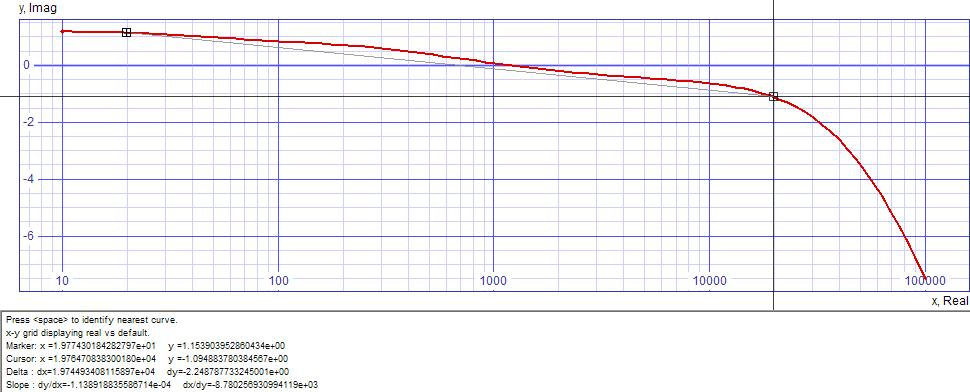SPICE Model, LSK170 SPICE Subckt Models

Optimizing RIAA network values in SPICE rids plot of waviness to slope down from 20Hz to 20kHz by only 1.60dB.  Calculations for adjustments are made in the following linear manner.  If measurement increases with increase in component value, calculate adjustment by:
 valuenew = valuecurrent x measurementtarget measurementobserved
Else if measurement decreases with increase in component value, calculate adjustment by:
 valuenew = valuecurrent x measurementobserved measurementtarget
If relationship is strictly linear, you can meet your target with one adjustment calculation.  If not, repeatly recalculate and resimulate until the target is met or close enough for your tolerance criteria.  To help you bend your graph as you desire you should note that frequency plot will slope down at a corresponding pole/zero pair (one in the recorded preequalization and the other in the equalization of your preamp) if any error is on the side of fpole<fzero (Tpole>Tzero) and slope up if any error is on the side of fpole>fzero (Tpole<Tzero).  You can make your sound bright or warm by your adjustments.

 Figure 5:  Frequency response of SPICE optimized circuit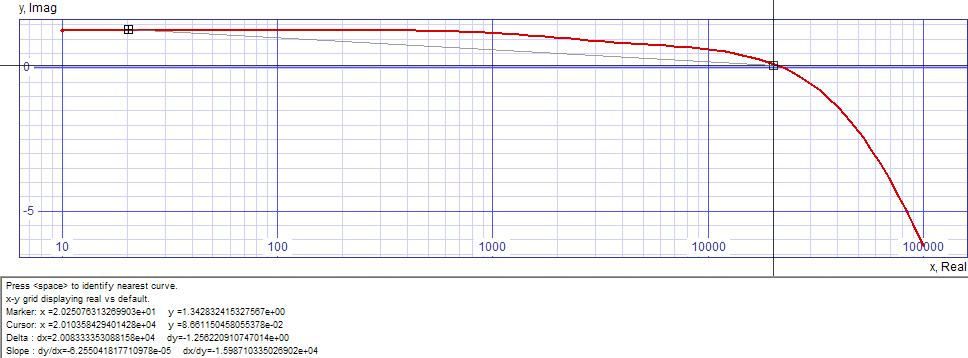SPICE Model, LSK170 SPICE Subckt Models

After careful rounding of component values plot slopes down from 20Hz to 20kHz by only 1.30dB.

 Figure 6:  Frequency response of final circuit after choosing standard component values.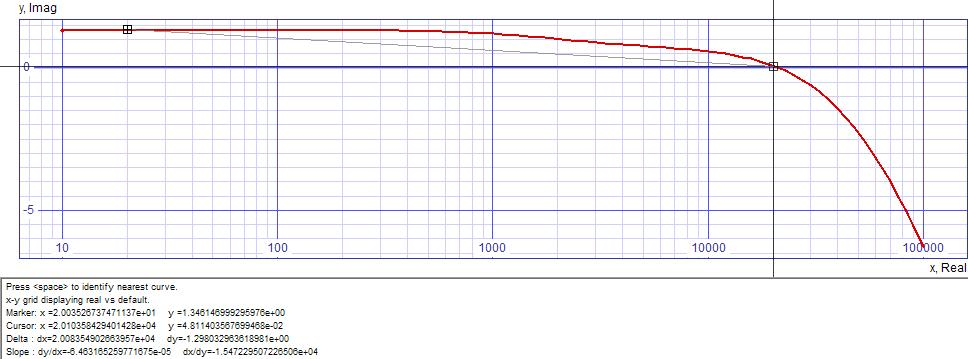SPICE Model, LSK170 SPICE Subckt Models

Higher than anticipated JFET transconductance may require raising supply voltage to prevent drain saturation.  Unexpected distortion of prototype may be aleviated by raising power supply voltage.

#### Other SPICE Findings

SNR = 102.98dB relative to 1Vrms output.  This would be relative to a 10mV cartridge output amplified by 40dB gain at 1kHz.  SNR will vary with cartridge output after you compensate with your volume control.

Fourier analysis for vout: (Distortion performance at 7.071mVpeak(5mVrms) and 1kHz and breakdown by harmonic)
Fourier analysis for vout:
No. Harmonics: 10, THD: 0.0178052 %, Gridsize: 200, Interpolation Degree: 1
 Harmonic Frequency Magnitude Phase Norm. Mag Norm. Phase -------- --------- --------- ----- --------- ----------- 0 0 0 0 0 0 1 1000 0.879288 -92.635 1 0 2 2000 0.000146834 -19.801 0.000166992 72.8345 3 3000 5.41796e-005 -38.326 6.16175e-005 54.3094 4 4000 3.23322e-006 -95.464 3.67709e-006 -2.8289 5 5000 1.56558e-006 -131.85 1.78051e-006 -39.21 6 6000 3.12765e-007 -88.13 3.55703e-007 4.50502 7 7000 1.21373e-006 -45.309 1.38036e-006 47.3267 8 8000 2.42554e-007 -80.335 2.75853e-007 12.3003 9 9000 7.75152e-007 58.3996 8.81568e-007 151.035

Fourier analysis for vout: (Distortion performance at 100Hz and proportionate level to 1kHz and breakdown by harmonic)
No. Harmonics: 10, THD: 0.0190292 %, Gridsize: 200, Interpolation Degree: 1
 Harmonic Frequency Magnitude Phase Norm. Mag Norm. Phase -------- --------- --------- ----- --------- ----------- 0 0 0 0 0 0 1 100 0.891848 -90.243 1 0 2 200 0.000169612 170.701 0.00019018 260.944 3 300 5.79251e-006 -75.974 6.49495e-006 14.2693 4 400 3.3312e-007 49.7897 3.73516e-007 140.033 5 500 2.1084e-007 94.8152 2.36408e-007 185.058 6 600 1.69153e-007 96.0689 1.89666e-007 186.312 7 700 1.44333e-007 96.9693 1.61835e-007 187.212 8 800 1.2705e-007 97.6061 1.42457e-007 187.849 9 900 1.13432e-007 98.8471 1.27188e-007 189.09

Fourier analysis for vout:  (Distortion performance at 10kHz and proportionate level to 1kHz and breakdown by harmonic)
No. Harmonics: 10, THD: 0.345553 %, Gridsize: 200, Interpolation Degree: 1
 Harmonic Frequency Magnitude Phase Norm.Mag Norm.Phase -------- --------- --------- ----- --------- ----------- 0 0 0 0 0 0 1 10000 0.821345 -102.31 1 0 2 20000 0.00283777 -107.17 0.00345502 -4.8656 3 30000 4.86413e-005 -79.038 5.92215e-005 23.2697 4 40000 2.79079e-006 168.487 3.39783e-006 270.794 5 50000 9.02139e-007 -74.142 1.09837e-006 28.1659 6 60000 9.62636e-007 -84.247 1.17202e-006 18.0606 7 70000 8.13981e-007 -83.821 9.91034e-007 18.4866 8 80000 7.11331e-007 -82.815 8.66056e-007 19.4925 9 90000 6.33031e-007 -81.892 7.70725e-007 20.4162

#### Miscellaneous Calculations

Now set coupling capacitor values C3 and C5 for best bass response:
Initially desire both highpass poles at 0.1Hz for nearly no affect at 20Hz.

Rpole = R6 || (rds/4) + R7 || R8 = 2kΩ || 20.479kΩ + 10MΩ || 442kΩ = 1.82206kΩ + 423.291kΩ = 425.113kΩ
 C3 = 1 2πfpoleRpole = 1 2π x 0.1Hz x 425.113kΩ = 3.74383µF
Round up to next 20% standard value.  Choose C3 = 3.9µF polyethylene.

Assuming a high impedance load (RL >= 10kΩ):
Rpole = Rout-Q6  + R14 || RL = 42.3682Ω + 100kΩ || 10kΩ = 9.13328kΩ
 C5 = 1 2πfpoleRpole = 1 2π x 0.1Hz x 9.13328kΩ = 174.258µF
Round up to next 10% standard value.  Choose C5 = 180µF electrolytic
If you want a polyethylene capacitor here choose C5 = 10µF and recalculate pole:
 fpole = 1 2πC5Rpole = 1 2π x 10µF x 9.13328kΩ = 1.74258Hz
Highpass response is only down -0.033dB at 20Hz with this choice of 0.1Hz and 1.74258Hz poles.

You may recalculate C5 if you want to drive 600Ω.

Calculate load current from power supplies VDD1, VDD2, and VDD3.
iDD1 = iQ1-BIAS x 4 + iR7-R8 = 2.551918mA x 4 + 48V/(10MΩ + 442kΩ)  = 10.2123mA
iDD2 = iQ6-BIAS = 2.03827mA
iDD3 = iQ6-BIAS = 5mA

#### Design Decisions Left to You

• R1 and C1 should be calculated per article Phono Termination Calculations and Calculator.
• This circuit and its analysis is only given as a design example; change everything if you like.
• A low noise 48V power supply.  The Line Level Class A Power Supply  presented earlier will require a design improvement to deliver 48V.  The LM317 used as a preregulator has a 40V limit on the input/output differential.  See the LM317 datasheet for ideas.
• Whether protection zeners (39V) should be used to protect from drain overvoltage.  (Connected from drain to source or ground.)  Load resistors may provide sufficient protection.
• Resistor wattage given for normal operation.  Decide whether more wattage is needed at power up.
• In spite of careful design, transistor parameter variations may require more adjustments during the prototyping process.  The specifications in this design are only valid if the JFETs match the SPICE model parameters.  ;=(

Document History
June 26, 2010  Created
July 3, 2010  Recalculated with 48V power supply and RIAA standard gain.
January 26, 2011
Removed personal comment at beginning.  Made minor symbol improvements.
March 26, 2011  Corrected numerical typo at end of first stage calculations not affecting their results and a misspelling in the SPICE results.